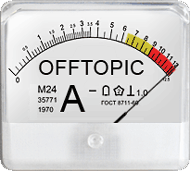## OT: Your signature [Bioanalytics]

Hi ElMaestro,

x=c("Foo", "Bar") b=data.frame(x) typeof(b[,1]) ##aha, integer? b[,1]+1 ##then let me add 1Lemme explain:

x <- c("Foo", "Bar") is.character(x)  TRUE b <- data.frame(x) is.character(b)  FALSE is.data.frame(b)  TRUE print(b)     x 1 Foo 2 Bar
So far, so good. What else?
str(b) 'data.frame':   2 obs. of  1 variable:  $x: Factor w/ 2 levels "Bar","Foo": 2 1 Implicitly you are using the default of data.frame() like here: c <- data.frame(x, stringsAsFactors=TRUE) Check #1 identical(c, b)  TRUE Check #2 c == b x [1,] TRUE [2,] TRUE See the point? typeof(b[, 1]) ##aha, integer?  "integer" Correct. Factors are always coded as integers internally. Check #3 is.factor(b[, 1])  TRUE Note also the output of str(b) above where factors are given in lexical order. However, since we defined x <- c("Foo", "Bar"), "Bar" gets the level 2 and "Foo" the level 1 in b. b[, 1]+1 ##then let me add 1  NA NA Warning message: In Ops.factor(b[, 1], 1) : ‘+’ not meaningful for factors Well roared, lion! On the other hand: d <- data.frame(x, stringsAsFactors=FALSE) print(d) x 1 Foo 2 Bar str(d) 'data.frame': 2 obs. of 1 variable:$ x: chr  "Foo" "Bar" typeof(c[, 1])  "character"
Is this what you expected?
d[, 1]+1 Error in c[, 1] + 1 : non-numeric argument to binary operator
Sure. Rubbish in, rubbish out.
Personally I prefer a straight error over a warning producing NAs.Dif-tor heh smusma 🖖 Довге життя Україна!Helmut SchützThe quality of responses received is directly proportional to the quality of the question asked. 🚮
Science QuotesIng. Helmut Schütz